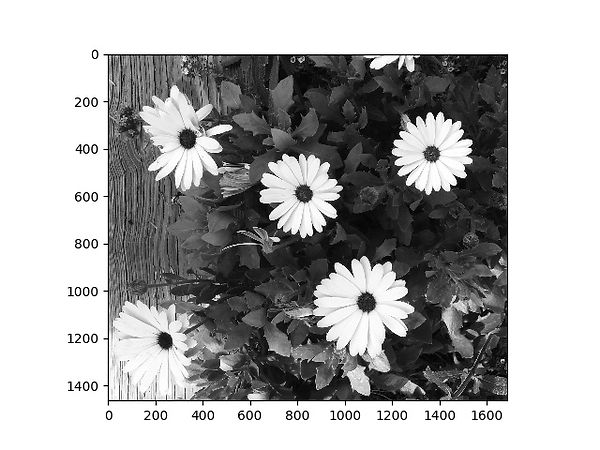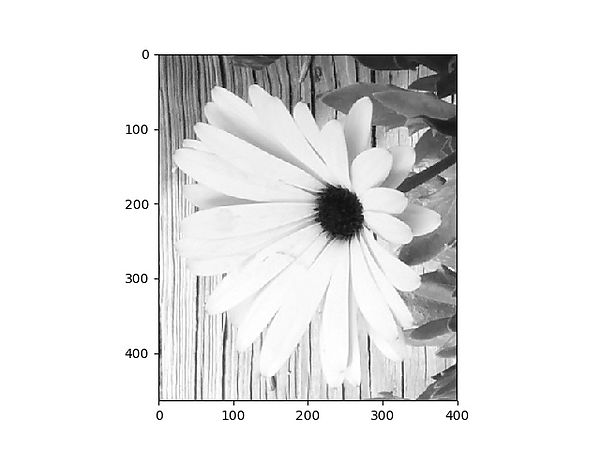top of page

# Summary

### Image features

Oftentimes, the types of images you'll analyze for a scientific purpose won't be in color. This might be because color is not important to the task at hand (e.g., if you’re tracking the motion of something under a microscope) or because the image is mapping some other quantity entirely (like an MRI, which maps signals coming from your tissues after a magnetic field is applied). Thus, image pixels do not necessarily have RGB values associated with each pixel. Instead, each pixel will be associated with a single value that denotes the amount of signal being measured (i.e., grayscale). In the case of photographs, the signal is light.

We will read the flowers image into Python again, but this time we will convert to grayscale. The code is nearly identical to what was shown previously, but we add an "as_gray=True" argument to the imread function call.

Note that when we print the shape of the image now, we get (1464, 1687) instead of (1464, 1687,3) because each pixel now corresponds to a single intensity value rather than an RGB color. The value is also normalized so that the maximum value corresponds to 1, since the computer does not have information about your units.

You should also now get a plot of the image in grayscale like the one below:Again, since the image is stored in Python as a NumPy array, we can select part of the image by using normal indexing. In the code above, the code "subimage = imagefile[1000:, :400]" will select all the pixels with y-positions above 1000 and x-positions up to 400 (i.e., the lower-left corner of the image. This is like cropping an image. The result is shown below. Note that the coordinates are now re-numbered so that (0,0) corresponds to the origin of the subimage.We can also identify the maximum (largest) and minimum (smallest) values of the image using np.max() and np.min(), respectively, each of which takes an array as an argument. We see that the maximum and minimum values are 1.0 and 0.00196, respectively. In this particular case, 1.0 corresponds to completely white, 0.0 corresponds to completely back, and values in-between correspond to shades of gray (larger values are lighter).

What is more useful is finding out where in the image these maximum and minimum values occur using the np.where() function, which returns all the values that meet a certain condition. For example, the command "max_yvals, max_xvals = np.where(imagefile==maxvalue)" will return the y and x coordinates of all the pixels in the image that are equal to the maximum value of the image. The locations of the pixels matching the minimum value can be found in a similar way.

Using matplotlib, we take our grayscale image and make a scatterplot of the locations of the brightest pixels (in blue) and the darkest pixels (in red). We see that the maximum pixel values can be found in the flowers, while the darkest pixels (see the red dot in the lower right corner) can be found in a shadow.The results above suggest that if we want to pick out the locations of the flowers, we can try selecting the brightest pixels through a command such as "upper_yvals, upper_xvals = np.where(imagefile>=0.9maxvalue)," which returns the coordinates of all the pixels with values that are at least 90% of the maximum value. We make a scatterplot of these coordinates in pink below. We see that this simple step actually does a surprisingly decent job of picking out the pixels belonging to the flowers, but it's not perfect - we don't get all of the pixels belonging to the flowers, and we also selected some of the pixels belonging to leaves. Nevertheless, this demonstrates something important: we can search for features in an image if we know roughly what values they have, and we can figure out what kind of feature a pixel belongs to by measuring its value. The next part of the lesson will explore some more sophisticated image processing techniques.1  #import image processing package

from skimage import io

4  imagefile = io.imread("Flowers.jpg", as_gray = True)

print("The shape of the image array is", imagefile.shape)

6

#import package to show the image

import matplotlib.pyplot as plt

9  fig1 = plt.figure()

10 plt.imshow(imagefile, cmap = 'gray')

11 plt.savefig("Flowers_grayscale.jpg")

12

13 import numpy as np

14 #make subimage

15 subimage = imagefile[1000:, :400]

16 f2 = plt.figure()

17 plt.imshow(subimage, cmap = 'gray')

18 plt.savefig("subimage.jpg")

19

20 #use NumPy to get basic information about the image

21 maxvalue = np.max(imagefile)

22 minvalue = np.min(imagefile)

23

24 print("The maximum value of the image is", maxvalue)

25 print("The minimum value of the image is", minvalue)

26

27 max_yvals, max_xvals = np.where(imagefile==maxvalue)

28 min_yvals, min_xvals = np.where(imagefile==minvalue)

29

30 #Figure out where minima and maxima are

31

32 f3 = plt.figure()

33 plt.ylim(ymin = 1464, ymax = 0)

34 plt.xlim(xmin = 0, xmax = 1687)

35 plt.imshow(imagefile, cmap = 'gray')

36 plt.scatter(max_xvals, max_yvals, s = 2, color = 'blue')

37 plt.scatter(min_xvals, min_yvals, s = 5, color = 'red')

38 plt.savefig("locations1.jpg")

39

40 upper_yvals, upper_xvals = np.where(imagefile>=0.9*maxvalue)

41

42 f4 = plt.figure()

43 plt.ylim(ymin = 1464, ymax = 0)

44 plt.xlim(xmin = 0, xmax = 1687)

45 plt.imshow(imagefile, cmap = 'gray')

46 plt.scatter(upper_xvals, upper_yvals, s = 1, color = 'pink')

47 plt.savefig("locations2.jpg")

bottom of page